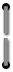# Appendix B

##### Combined Arithmetic Processes
The HP-80 performs combined arithmetic operations—serial, mixed and chain calculations—with ease. If you read Appendix A (Design Concepts) you now know how the operational stack works and can apply that knowledge to the following examples.

### Automatic Doubling, Squaring

If you want to double or square a number, it’s not necessary to press that number twice. Merely enter the number, save it and press+to double or×to square the number. For example, to double 3,
Enter:  See Displayed:

 3SAVE+6
3 doubled
The following illustrates the data flow in the stack.

 Press See Remarks 3 3. 3 is keyed into X.SAVE 3.00 3 is loaded into X and Y.+6.00 Contents of X and Y are added; result (6) is loaded into X.

To square 3,
Enter:  See Displayed:

 3SAVE×9
3 squared
Again in the stack,

 Press See Remarks 3 3. 3 is keyed into X.SAVE 3.00 3 is loaded into X and Y.×9.00 Contents of X and Y are multiplied and the product (9) is loaded into X.55